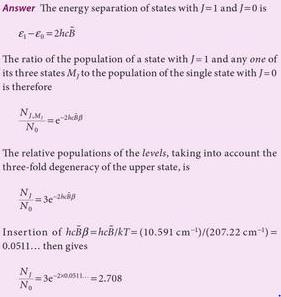Courses

# Atomic Structure & Quantum

## 60 Questions MCQ Test MOCK Test Series for IIT JAM Chemistry | Atomic Structure & Quantum

Description
This mock test of Atomic Structure & Quantum for Chemistry helps you for every Chemistry entrance exam. This contains 60 Multiple Choice Questions for Chemistry Atomic Structure & Quantum (mcq) to study with solutions a complete question bank. The solved questions answers in this Atomic Structure & Quantum quiz give you a good mix of easy questions and tough questions. Chemistry students definitely take this Atomic Structure & Quantum exercise for a better result in the exam. You can find other Atomic Structure & Quantum extra questions, long questions & short questions for Chemistry on EduRev as well by searching above.
QUESTION: 1

### The vibrational energy levels, v11 = 0 and v1 = 1 of a diatomic molecule are separated by 2143 cm-1. Its Anharmonicity (ωe Xe ) is 14 cm-1. The values of ωe (in cm-1) and first overtone (cm-1) of this molecules are respectively.

Solution: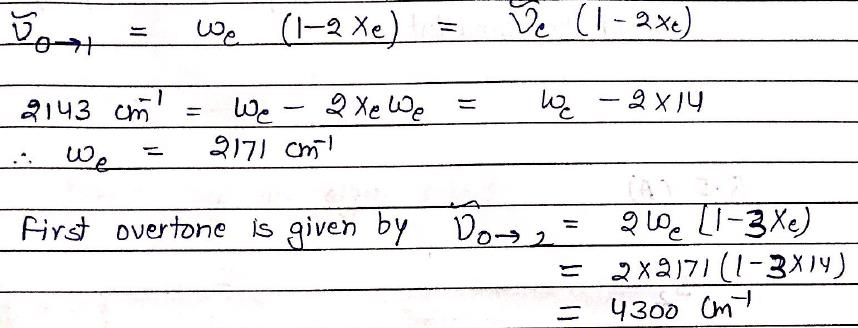QUESTION: 2

### The bond that gives the most intense band in the infrared spectrum for its stretching vibration is:

Solution: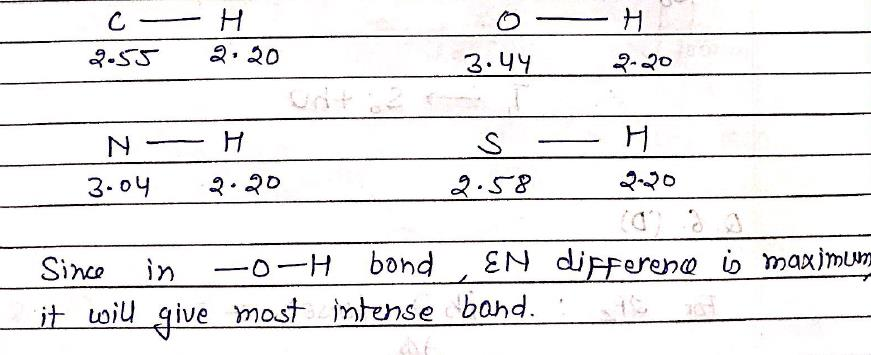QUESTION: 3

### In NMR spectroscopy, the product of the nuclear ‘g’ factor (gN), the Nuclear Magneton βN and the magnetic field strength (B0) gives the:

Solution: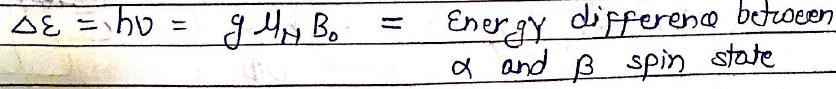QUESTION: 4

The molecule with the smallest rotational constant (in the microwave spectrum) among the following is:

Solution: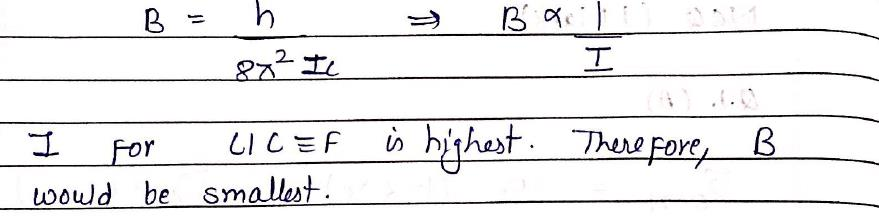QUESTION: 5

"Phosphorescence" is represented as:

Solution: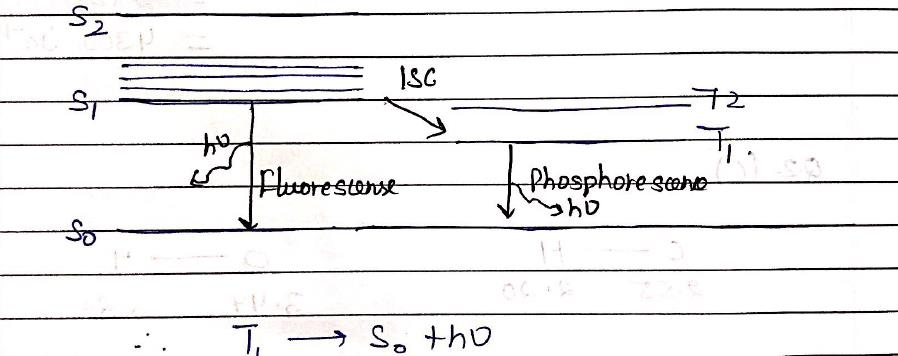QUESTION: 6

The following is true of 2px, 2py and 2pz orbitals of a H-atom:

Solution: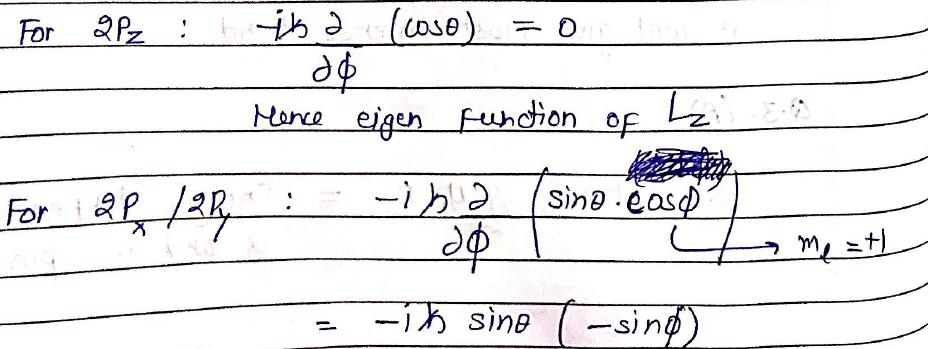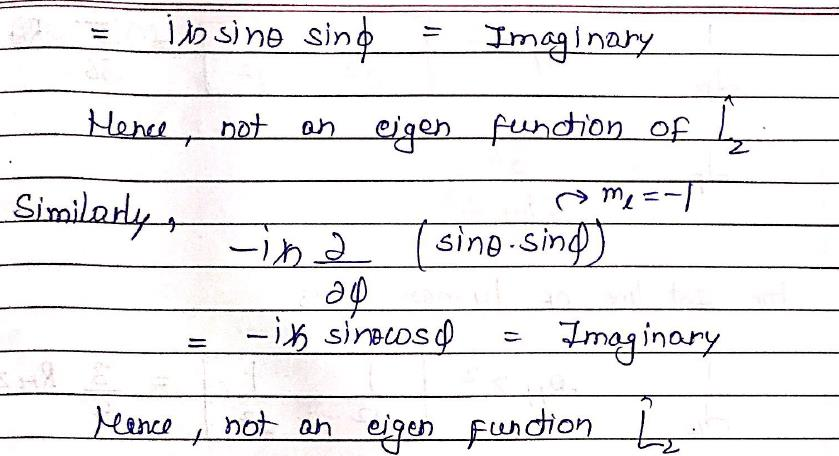QUESTION: 7

The wave function for a quantum mechanical particle in a 1-dimetional box of length ‘a’ is given as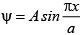. The value of ‘A’ for a box of length 200 nm is:

Solution: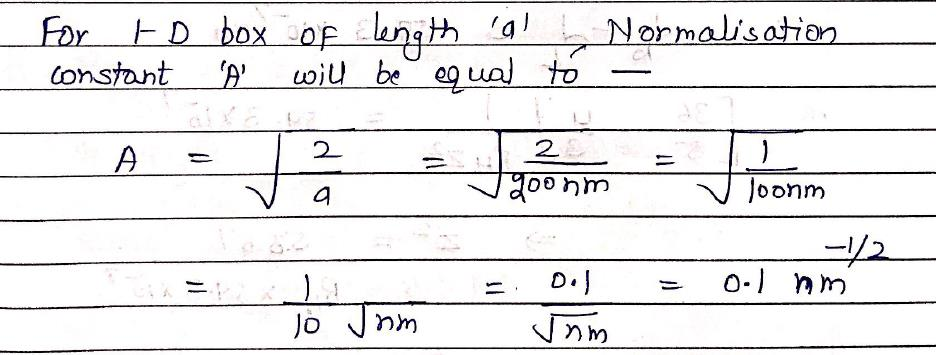QUESTION: 8

Which hydrogenic atom ion has the wavelength difference between first line of Balmer and Lymen Series equal to 59.3 nm? (RH = 109678 cm–1).

Solution: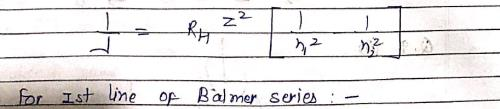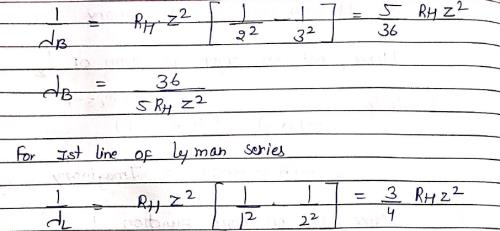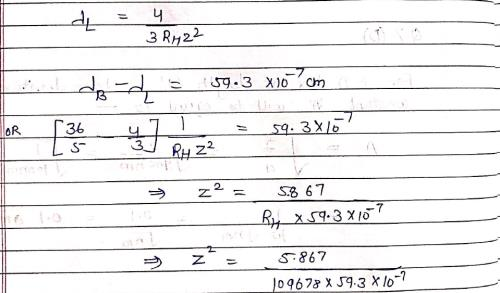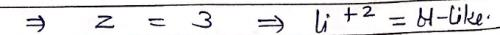QUESTION: 9

dz2 orbital has:

Solution: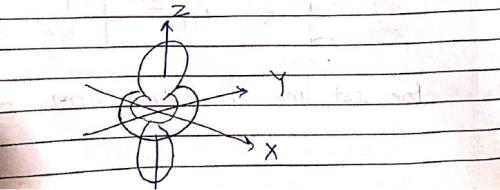QUESTION: 10

The commutator [X3, px] is equal to:

Solution: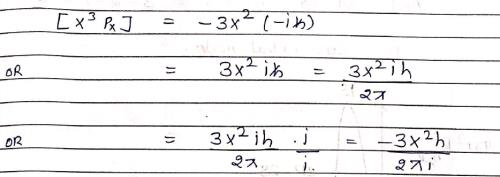QUESTION: 11

Which of the following is true for the radial part of the H atom wave function Rn, l(r) (n-principal quantum number and l-azimuthal quantum number) and the nodes associated with them:

Solution: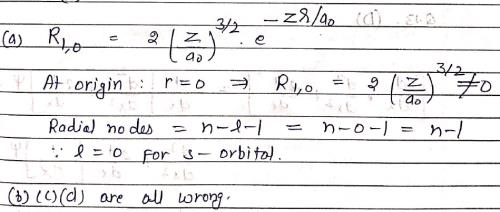QUESTION: 12

Which of the following radial distribution graphs correspond to l = 2 for H atom for the least value of ‘n’ for which l = 2 is allowed?

Solution: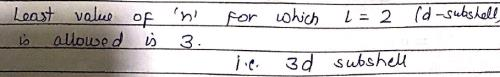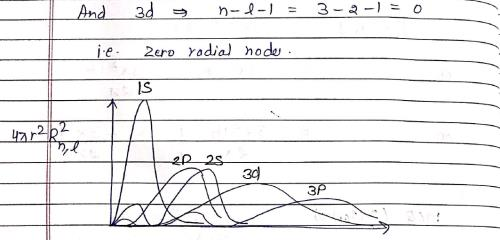QUESTION: 13

The value of the commutation of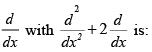Solution: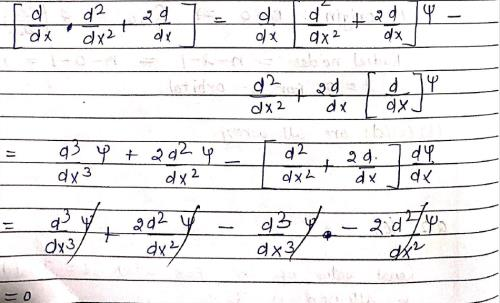QUESTION: 14

The difference between nth and (n + 1)th Bohr’s radius of H atom is equal to its (n –1)th Bohr’s radius the value of n is:

Solution: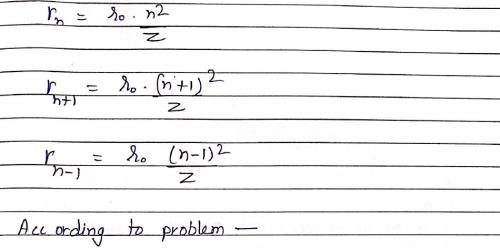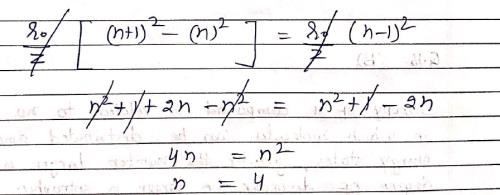QUESTION: 15

Consider the following statements: (I) Electron density in the XY plane in 3d x2 -y2 orbital is zero (II) Electron density in the XY plane in 3dz2 orbital is zero (III) 2s orbital has one nodal surface (IV) For 2pz orbital, YZ is the nodal plane, Which are the correct statements

Solution: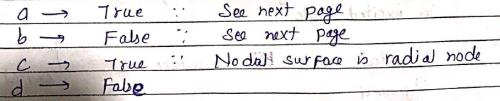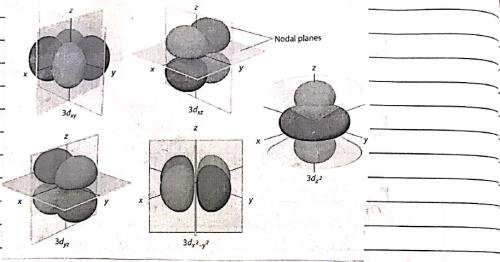QUESTION: 16

At room temperature, which molecule has maximum rotational entropy?

Solution: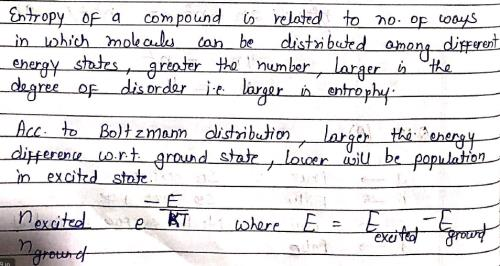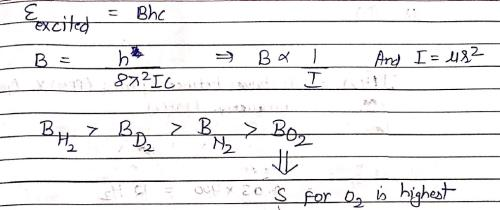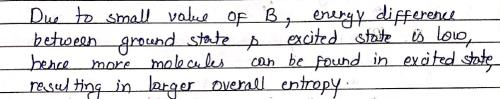QUESTION: 17

In IR spectrum of [Co(CN)5H]3–, the Co—H stretching is observed at 1840 cm–1. The Co—D stretch in [Co(CN)5D]3– will appear at nearly:

Solution: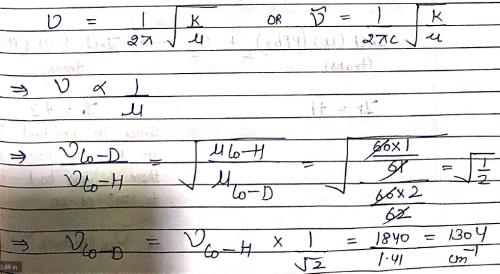QUESTION: 18

In 400 MHz 1H MNR spectrum of an organic compound exhibited a doublet. The two lines of the doublet are at δ = 2.35 and 2.38 ppm. The coupling constant (J) value is:

Solution: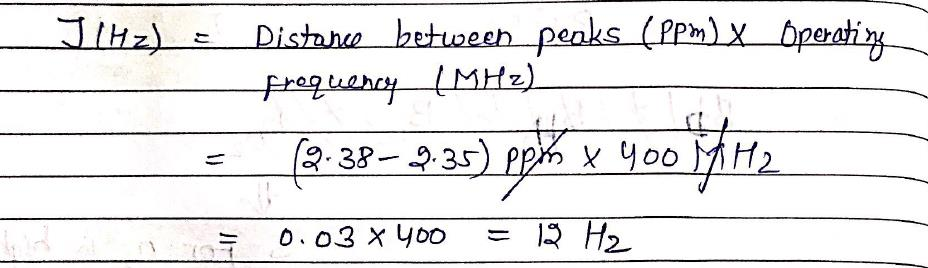QUESTION: 19

The spectroscopic technique, by which ground state dissociation energies of diatomic molecules can be estimated is:

Solution: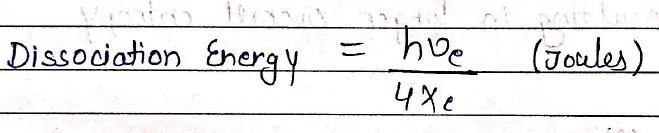QUESTION: 20

For reaction,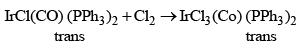The correct observation is:

Solution: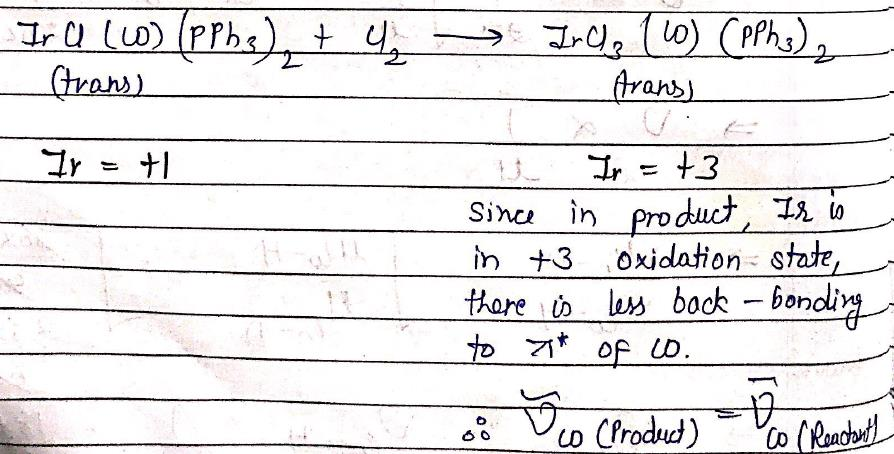QUESTION: 21

Which is/are true:

Solution: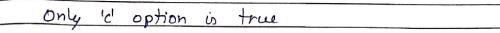QUESTION: 22

For an electron in a hydrogen atom, the wave function, ψ is proportional to exp(-r/a0), where a0 is the Bohr’s radius. What is the ratio of the probability of finding the electron at the nucleus to the probability of finding it at a0:

Solution: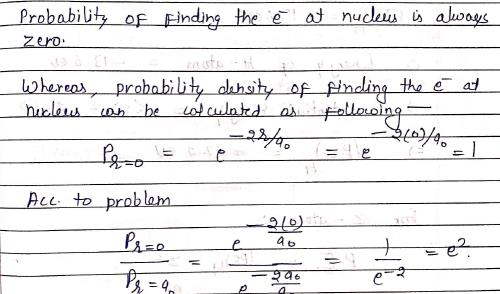QUESTION: 23

Which one of the following is an acceptable wave function for an electron in the hydrogen atom? (r, θ, φ) denote the spherical polar co-ordinates and N is a suitable constant which you are allowed to choose suitably:

Solution: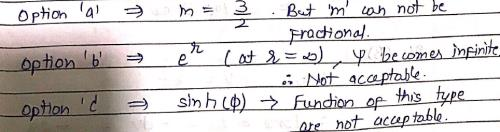QUESTION: 24

If the principal quantum number n = 6, the correct sequence of filling of electrons will be:

Solution:
QUESTION: 25

The ionization energy of H atom in its ground state is approximately 13.6 eV. The potential energy of He+, in its ground state is approximately:

Solution: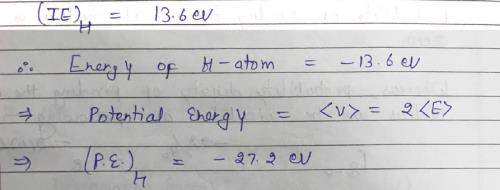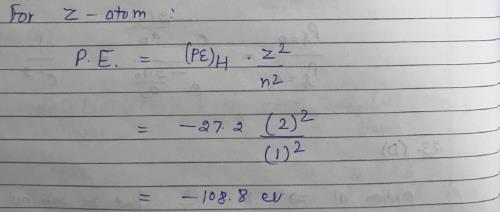QUESTION: 26

Vibrations of a diatomic molecule are usually modelled by a harmonic potential. If the potential is given by X2, the correct statement is:

Solution: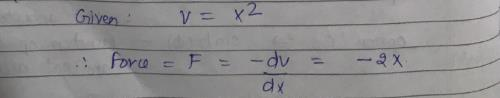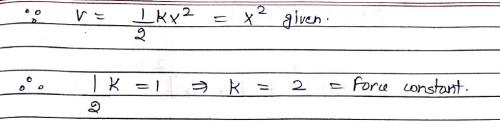QUESTION: 27

1H NMR spectrum of an organic compound recorded on a 500 MHz spectrometer showed a quartet with line positions at 1759, 1753, 1747, 1741 Hz. Chemical shift (s) and coupling constant (Hz) of the quartet are:

Solution: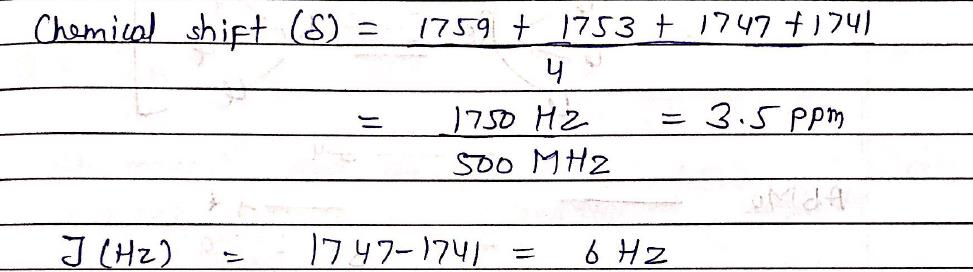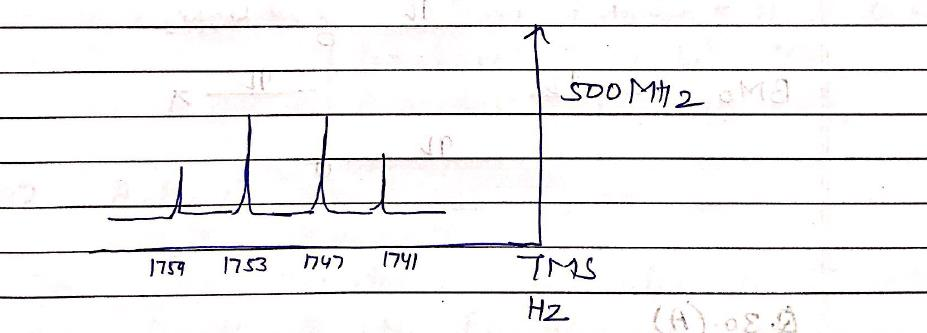QUESTION: 28

Which of the following spectroscopic techniques will be useful to distinguish between M-SCN and M-NCS binding modes?

Solution: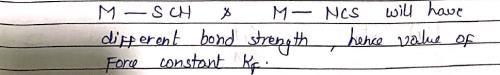QUESTION: 29

The inters bond at 15000 cm–1 in UV visible spectrum of [Bu4N]2 Re2Cl8 is due to transition:

Solution: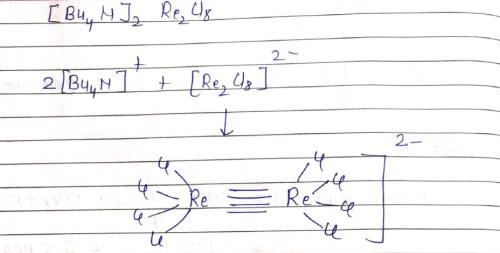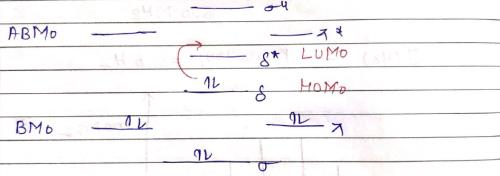QUESTION: 30

The 1H NMR frequency at 1.07 T is 42.4 MHz. If gyromagnetic ratio of 1H and 13C are 2.7 × 107 and 6.75 × 107 T–1 S–1, respectively, what will be the 13C frequency at 1.07 T:?

Solution: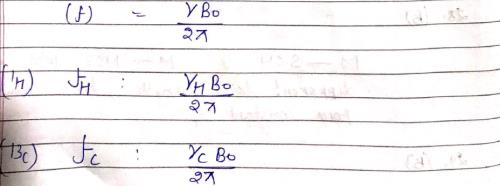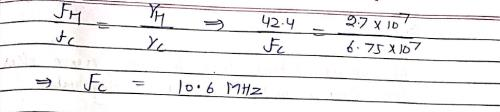*Multiple options can be correct
QUESTION: 31

Which of the molecule(s) has/have a pure rotational spectrum:

Solution: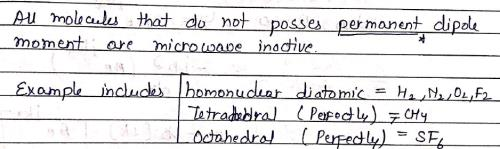*Multiple options can be correct
QUESTION: 32

Which of the molecule(s) is/are infrared active:

Solution: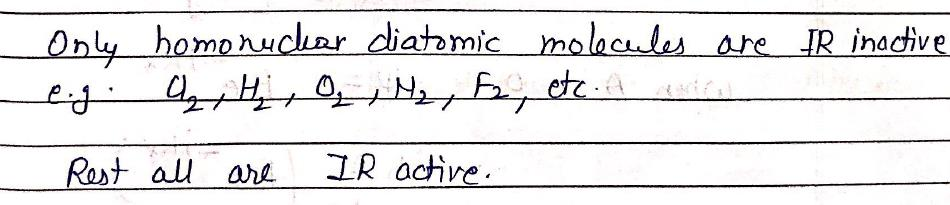*Multiple options can be correct
QUESTION: 33

The set of allowed electronic transition among following is:

Solution: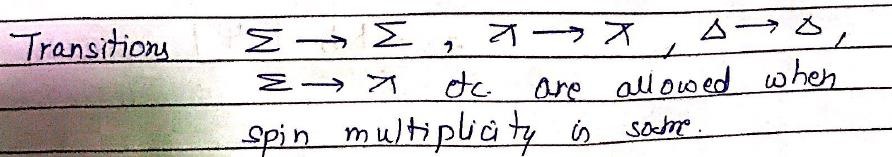*Multiple options can be correct
QUESTION: 34

Which is the following statement is/are wrong:

Solution: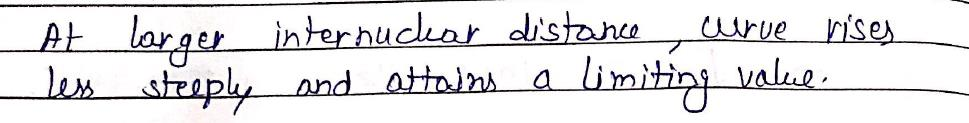*Multiple options can be correct
QUESTION: 35

Choose the correct statements among the following:

Solution:
*Multiple options can be correct
QUESTION: 36

The linear momentum of a particle describe by the wavefunction ψ(x) = Aeikx + Be-ikx; then which of the following statement(s) is/are correct:

Solution: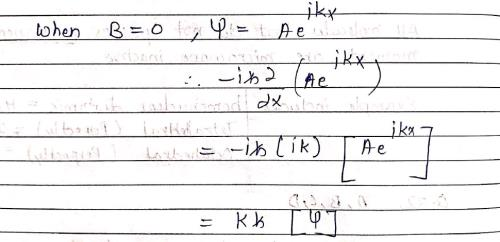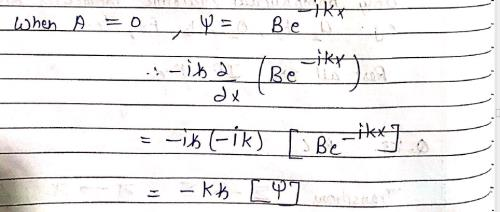*Multiple options can be correct
QUESTION: 37

Which of the following graph(s) for wave function is/are unacceptable:

Solution: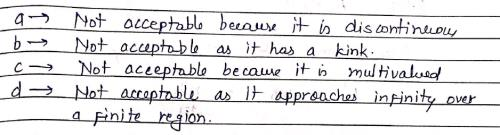*Multiple options can be correct
QUESTION: 38

Which of the following statements is/are correct?

Solution: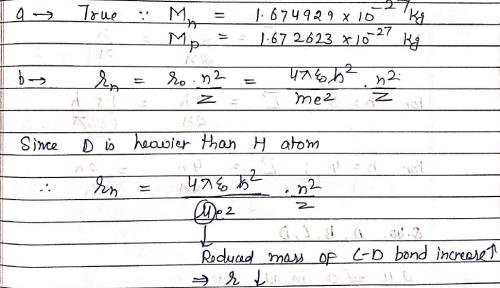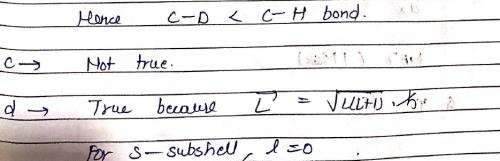*Multiple options can be correct
QUESTION: 39

According to Bohr’s theory, the angular momentum of electron can have the value(s):

Solution: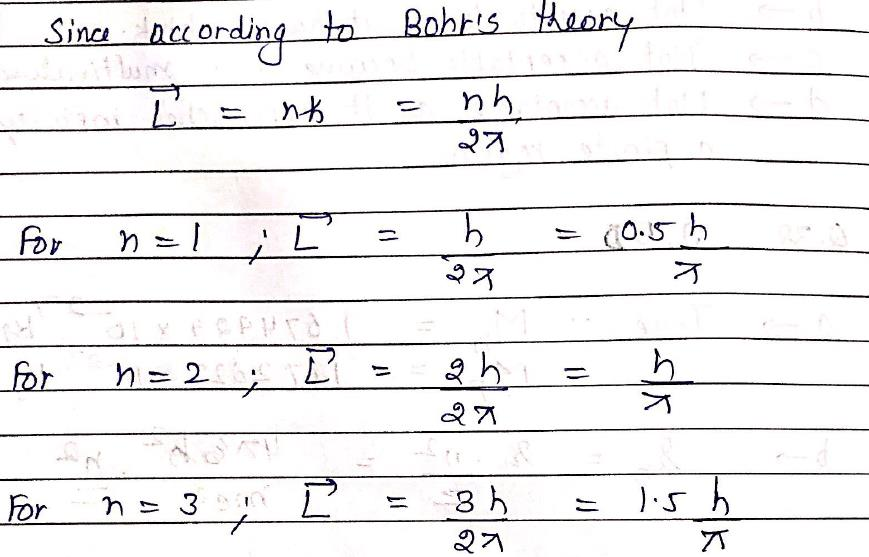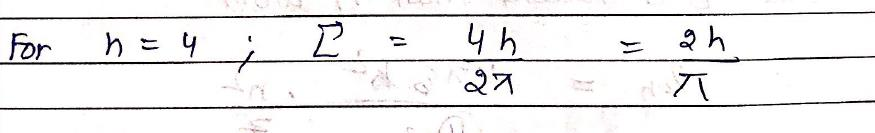*Multiple options can be correct
QUESTION: 40

Which of the following vibrational mode(s) is/are IR active:

Solution: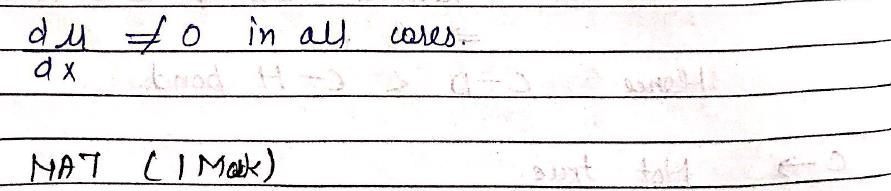*Answer can only contain numeric values
QUESTION: 41

In an atom, the total number of electrons having quantum numbers n = 4, |Ml| = 1 and ms = –1/2 is:

Solution: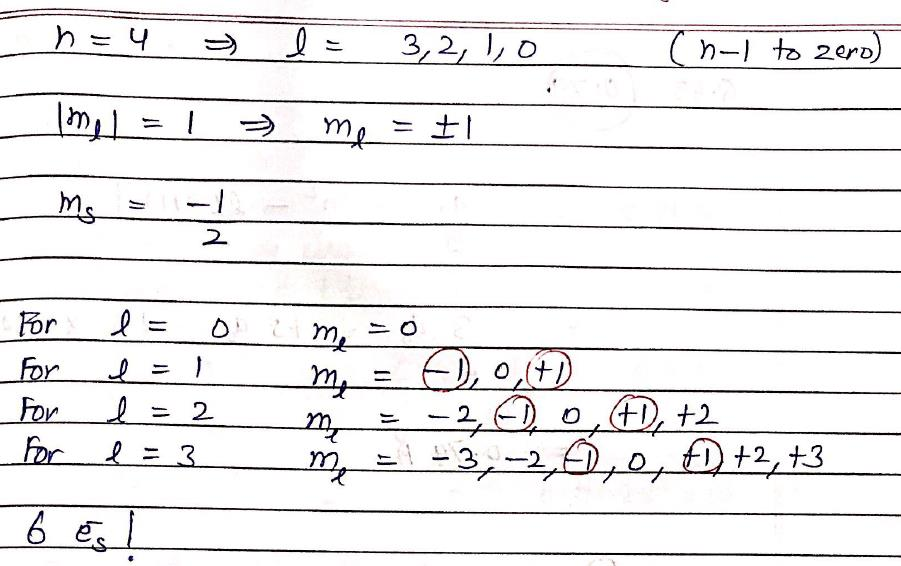*Answer can only contain numeric values
QUESTION: 42

A higher energy wave function of the electron in a nanotube is described by the normalized wave function (2/L)1/2sin(2πx/L). What is the percentage probability of finding the electron between x = L/4 and x = L/2?

Solution: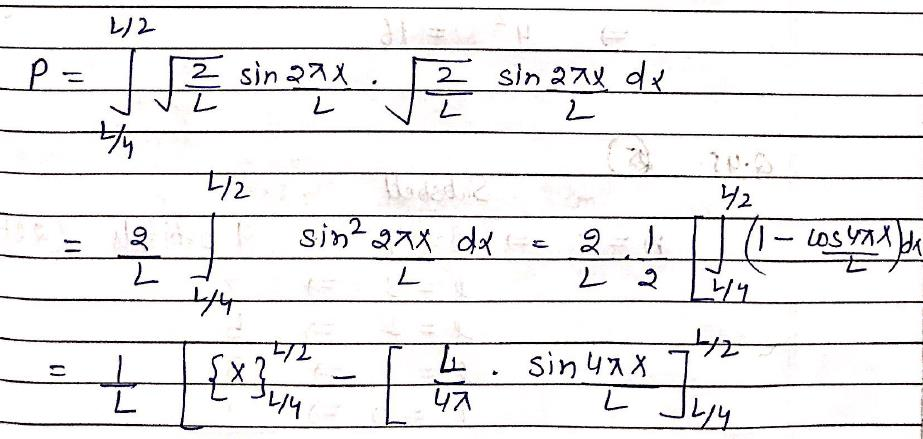*Answer can only contain numeric values
QUESTION: 43

Calculate the average value of radius (rounded up to two places, in Å) ‘r’ in 1s state of H-atom
described by wave function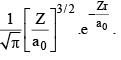Solution: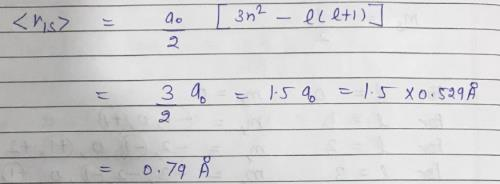*Answer can only contain numeric values
QUESTION: 44

What would be degeneracy of H-atom corresponding to n = 4?

Solution: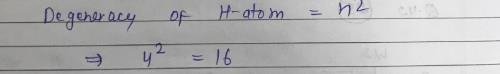*Answer can only contain numeric values
QUESTION: 45

The total number of orbitals associated with the principal quantum number 5 would be?

Solution: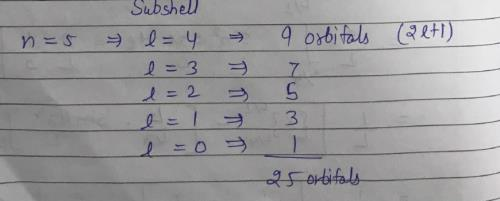*Answer can only contain numeric values
QUESTION: 46

The molar extinction coefficient of B (MW = 180) is 4 × 103 L mol–1 cm–1. One litre solution of C which contains 0.1358 g pharmaceutical preparations of B, shows an absorbance of 0.411 in a 1 cm quartz cell. The percentage (w/w) of B in pharmaceutical preparation is [rounded up to two decimal places]:

Solution: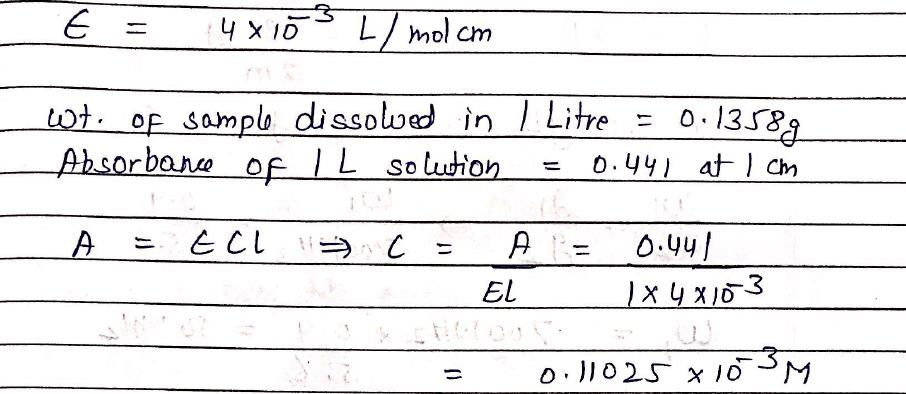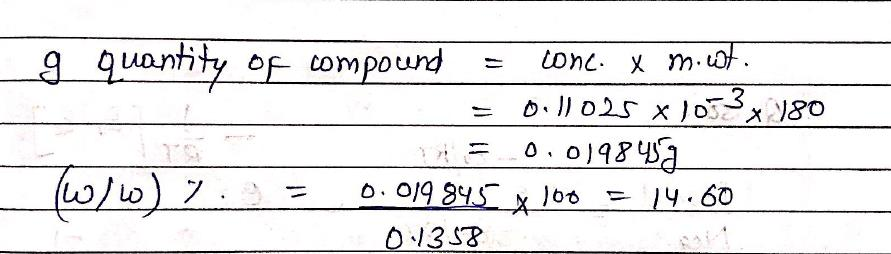*Answer can only contain numeric values
QUESTION: 47

The following data were obtained from vibrational fine structure in the vibronic spectrum of a diatomic Molecule: We = 512 cm–1, WeXe = 8 cm–1 Where We = energy associated with natural frequency of vibration Xe = Anharmonicity constant ​The dissociation energy (Dc) of molecule is (cm–

Solution: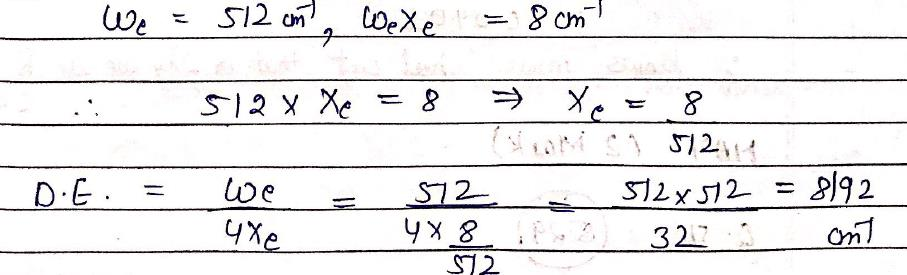*Answer can only contain numeric values
QUESTION: 48

Number of rotational symmetry axes for triclinic crystal system is:

Solution: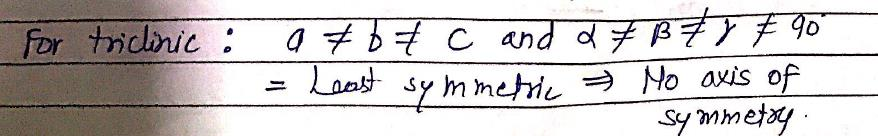*Answer can only contain numeric values
QUESTION: 49

The nuclear g-factor of 1H and 14N are 5.6 and 0.40 respectively. If the magnetic field in an NMR spectrometer is set such that the proton resonates at 700 MHz, the 14N nucleus would resonate at (MHz)? [rounded up to first decimal place]

Solution: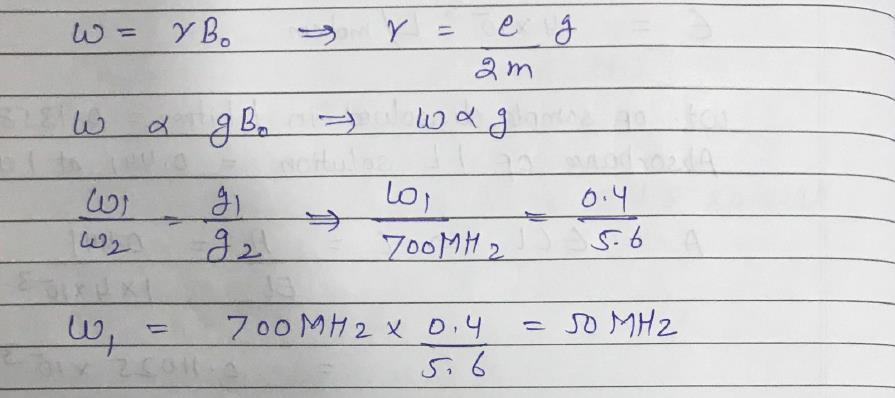*Answer can only contain numeric values
QUESTION: 50

Methylcyclohexane molecules may exist in one of the two conformation with the methyl group in either equatorial or axial position. The equatorial form is lower in energy with the axial form by 6kJ/mol. At a temp of 300 K, this difference in energy implies that relative population of molecules in axial and equatorial state is? [R = 8.314JK-1mol-1 rounded up to three decimal place]

Solution: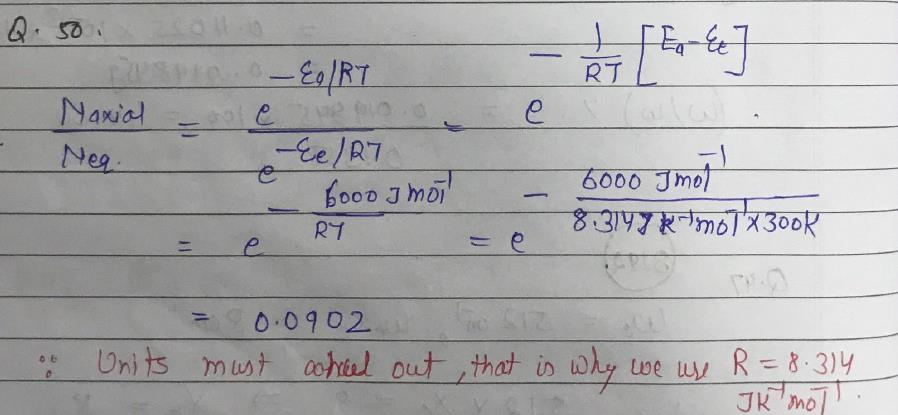*Answer can only contain numeric values
QUESTION: 51

Calculate the most probable radius at which an electron will be found when it occupies a 1s orbital of a Ne9+ (in pm, rounded up to two decimal place):

Solution: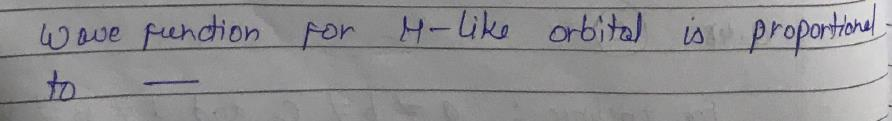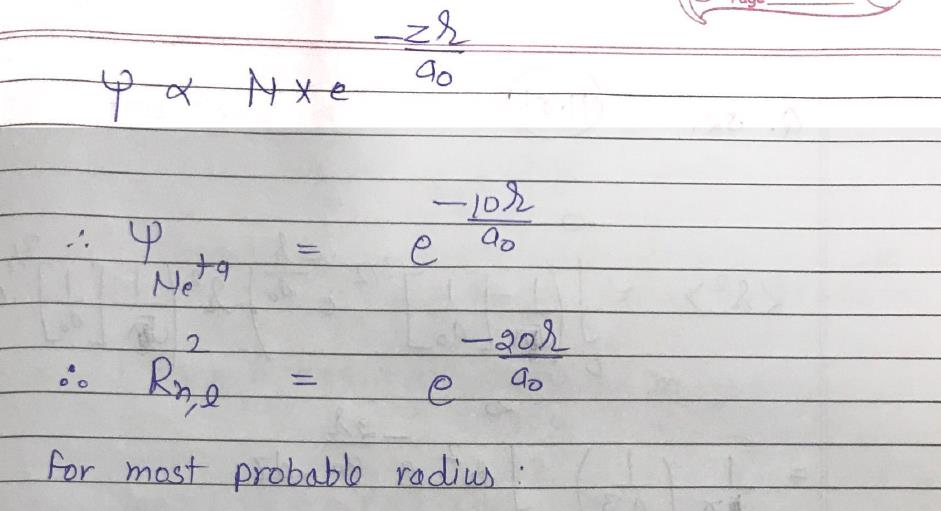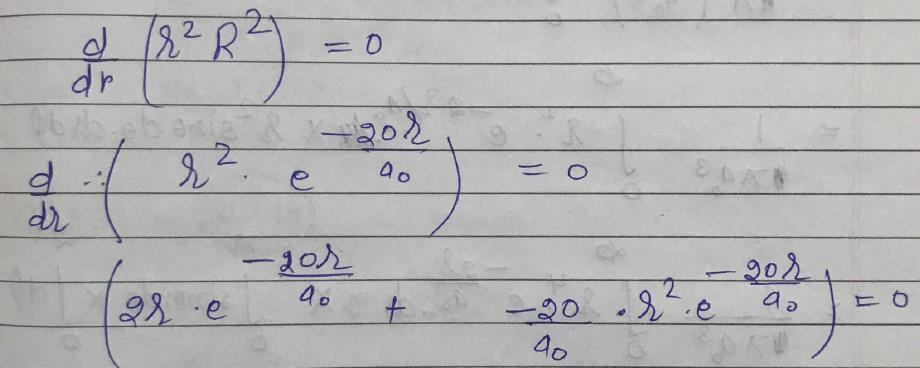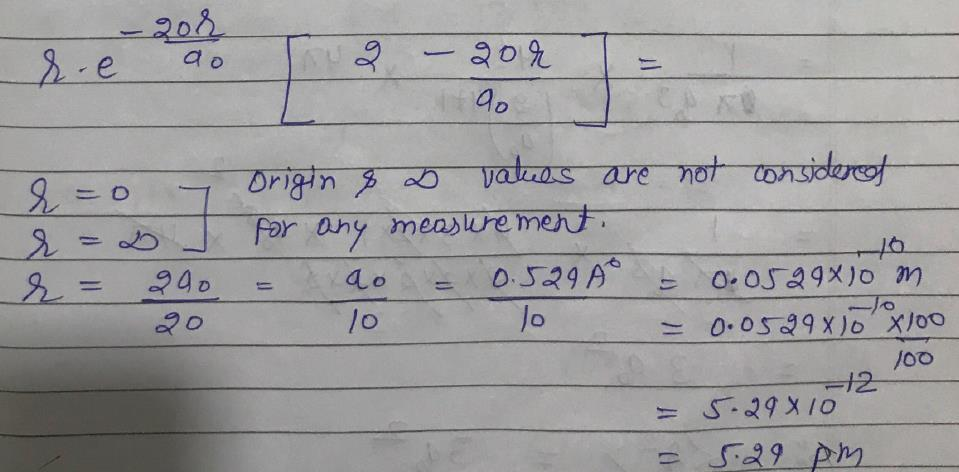*Answer can only contain numeric values
QUESTION: 52

Evaluate the root mean square distance, (r2)1/2 of the electron from the nucleus in the hydrogen
atom described by the wave function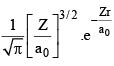[in pm rounded up to two decimal place]

Solution: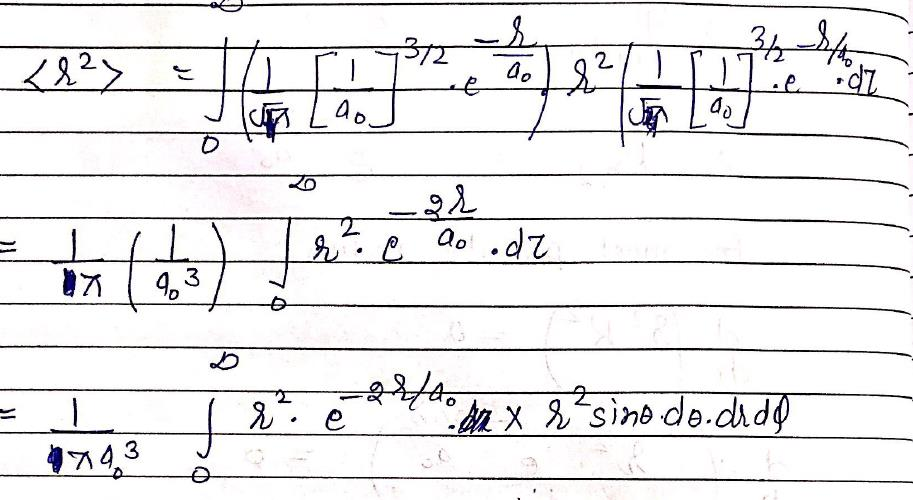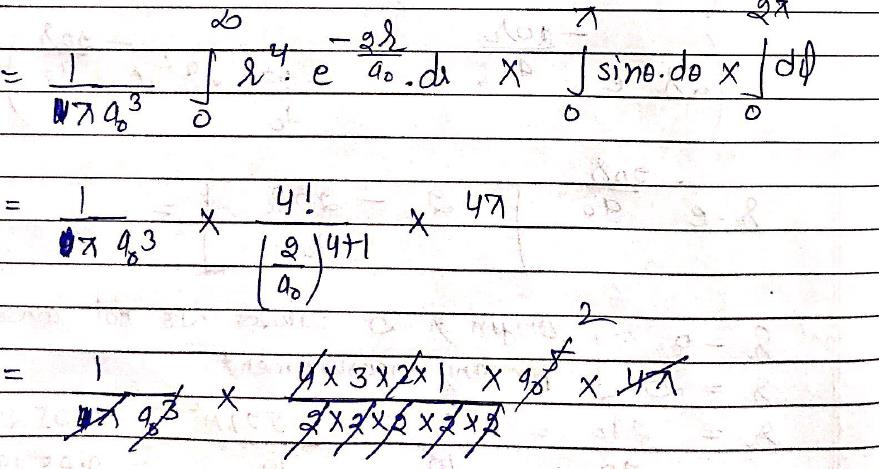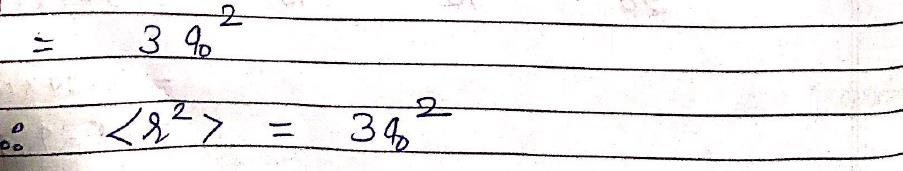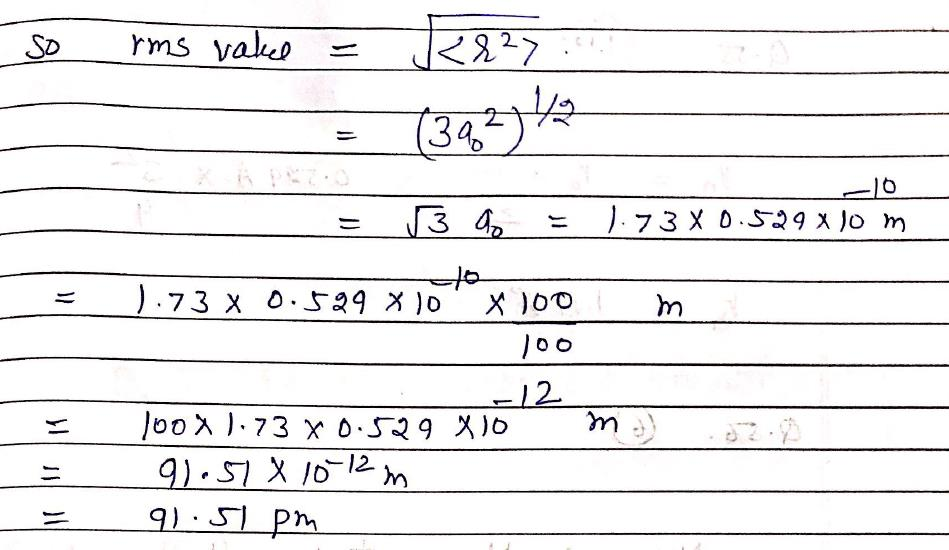*Answer can only contain numeric values
QUESTION: 53

Ground state energy of H-atom is –13.598 eV. The expectation value of Potential energy (in eV) would be? [rounded up to two decimal place]

Solution: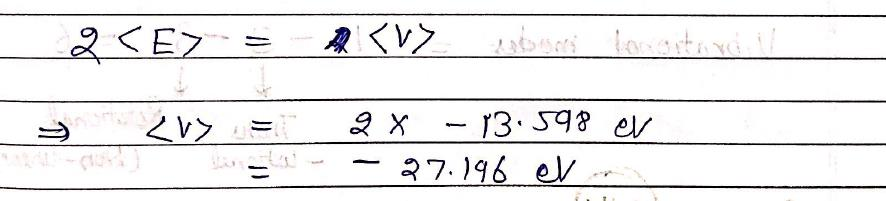*Answer can only contain numeric values
QUESTION: 54

What would be the degeneracy of 5th energy level in 3D box of length ‘L’?

Solution: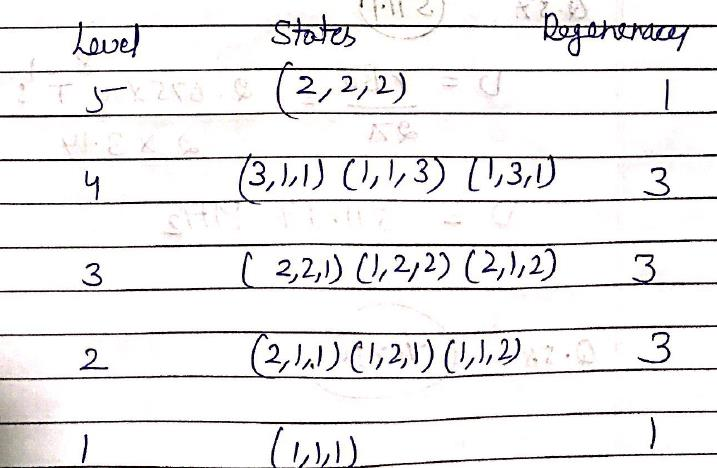*Answer can only contain numeric values
QUESTION: 55

What would be the 3rd radii (Å) of Be+3? [rounded up to two decimal place]

Solution: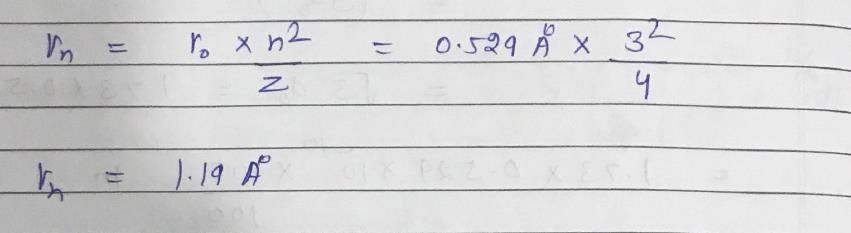*Answer can only contain numeric values
QUESTION: 56

The number of IR active vibrational modes is ammonia is?

Solution: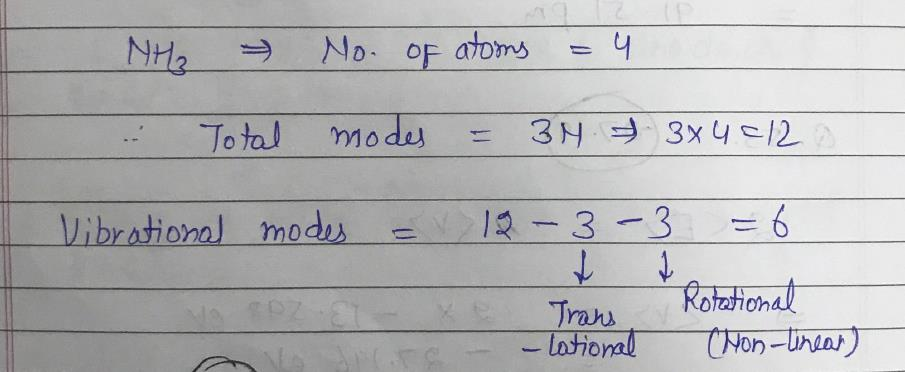*Answer can only contain numeric values
QUESTION: 57

Calculate the frequency (in MHz, up to 2 decimal place) at which radiation comes in to resonance with proton (I = ½) spins in a 12 T magnetic field? (Given: γN = 2.675 × 108T–1s–1) [rounded up to first decimal place]

Solution: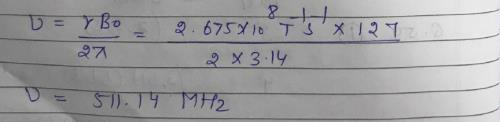*Answer can only contain numeric values
QUESTION: 58

Absorption spectrum of O2 shows a vibrational structure that becomes continuum at 56875 cm–1. At the continuum, it dissociates into one ground state atom (Og) and one excited state atom (Oe). The energy difference between Oe and Og is 15125 cm–1. The dissociation energy (cm–1) of G.S. O2 is:

Solution: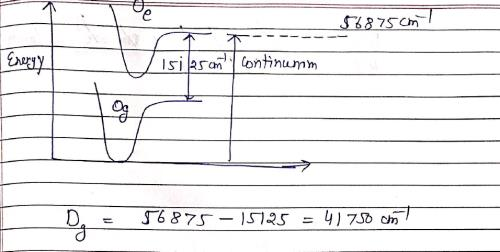*Answer can only contain numeric values
QUESTION: 59

What is the shift of the resonance from TMS of a group of nuclei with δ = 3.50 and an operating frequency of 350 MHz (in kHz)? [rounded up to two decimal place]

Solution: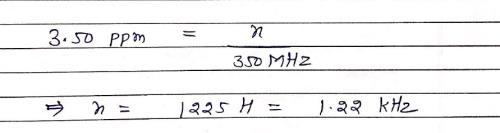*Answer can only contain numeric values
QUESTION: 60

Calculate the relative populations of the J = 1 and J = 0 rotational states of HCl at 25°C? [up to 2 decimal place] (Given: BHCl = 10.591 cm–1) [rounded up to three decimal place]

Solution: